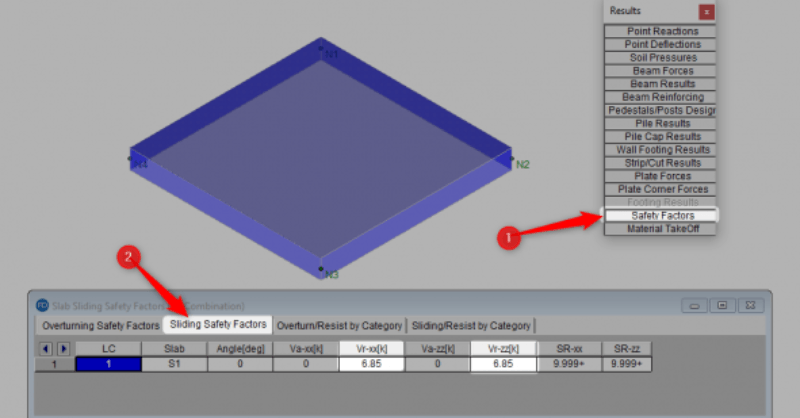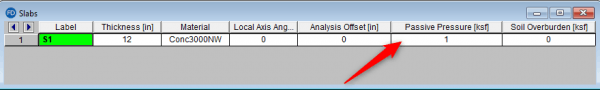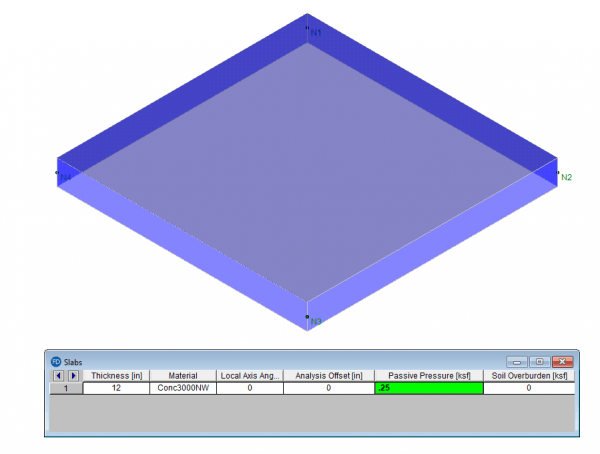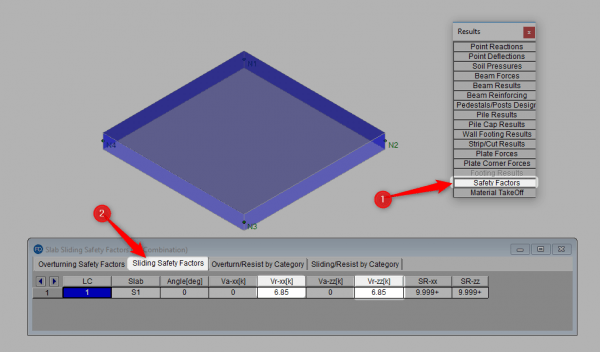RISAFoundation v11.0 now includes the added ability to enter a passive pressure for slabs. The passive pressure represents soil pressure along the perimeter of the slab and will contribute to the sliding resistance of the slab.RISAFoundation will multiply the passive pressure by the vertical area along the slab’s perimeter in both the slab’s local z and local x direction. This passive force will then be added to the sliding resistance due to friction in the Safety Factors spreadsheet.

In the below example we have a 10’ x 10’ x 1’-0” slab and we have set a passive pressure of 0.25 ksf.The sliding resistance due to passive pressure in one direction would therefore be equal to 0.25 ksf x 10’ width x 1’ depth = 2.5 kips. This would be added to the sliding resistance due to friction which is equal to 0.145 kcf x 10’ x 10’ x 1’ x 0.3 = 4.35 kips. Total resistance = 2.5 kips + 4.35 kips = 6.85 kips. We can confirm this within the Safety Factors spreadsheet.